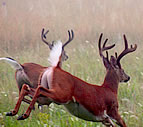×

# denominator

4 ENTRIES FOUND:
denominator /dɪˈnɑːməˌneɪtɚ/ noun
plural denominators
denominator
/dɪˈnɑːməˌneɪtɚ/
noun
plural denominators
Learner's definition of DENOMINATOR
[count] mathematics
: the number in a fraction that is below the line and that divides the number above the line